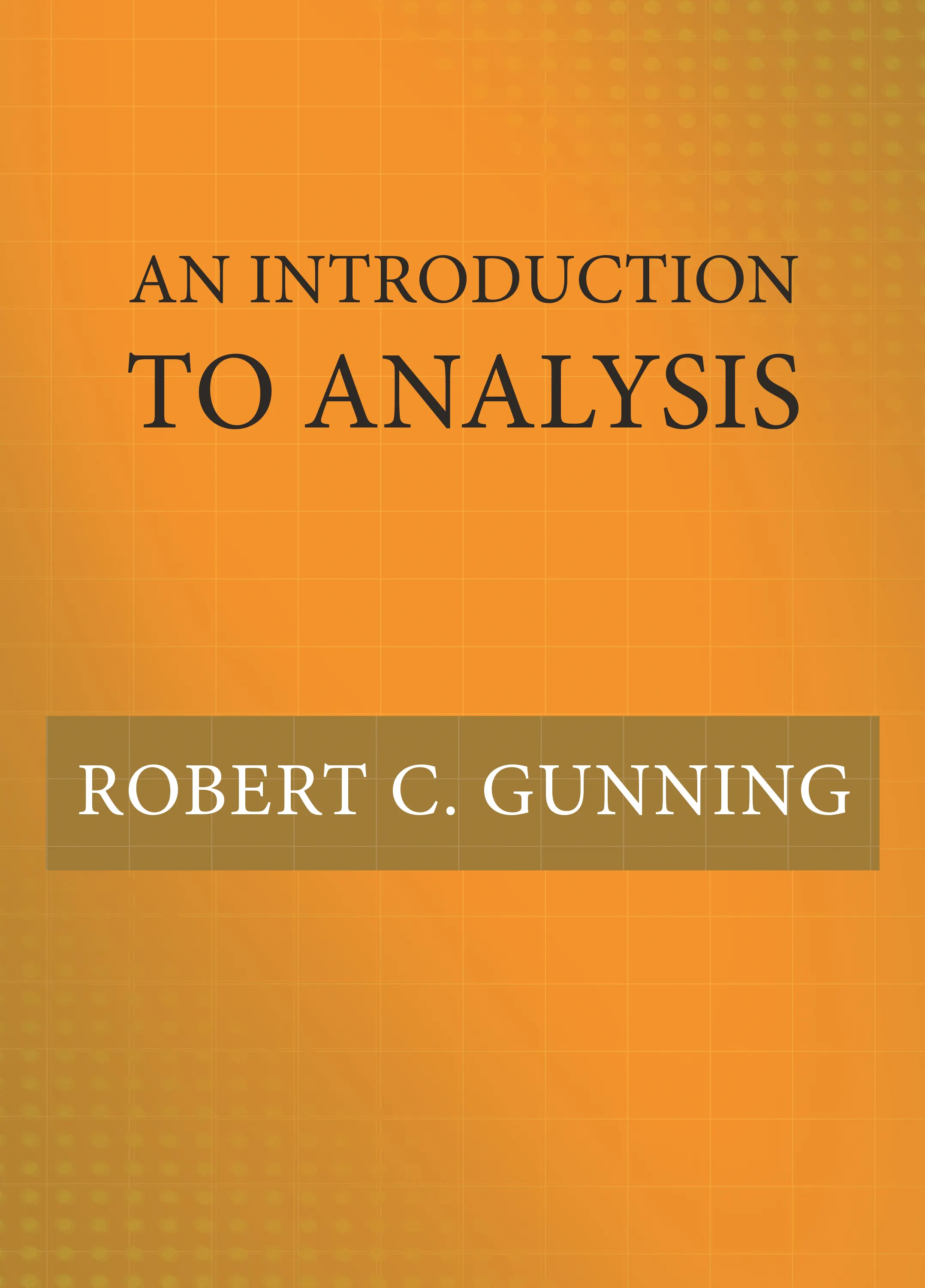#### An Introduction to Analysis

Robert C. Gunning

##### ebooks

Many of our ebooks are available for purchase from these online vendors:

Many of our ebooks are available through library electronic resources including these platforms:

# An Introduction to Analysis

An essential undergraduate textbook on algebra, topology, and calculus## Hardcover

Price:
\$80.00 / £62.00
ISBN:
Published:
Mar 20, 2018
2018
Pages:
384
Size:
7 x 10 in.
Illus:
25 b/w illus.

An Introduction to Analysis is an essential primer on basic results in algebra, topology, and calculus for undergraduate students considering advanced degrees in mathematics. Ideal for use in a one-year course, this unique textbook also introduces students to rigorous proofs and formal mathematical writing — skills they need to excel.

With a range of problems throughout, An Introduction to Analysis treats n-dimensional calculus from the beginning—differentiation, the Riemann integral, series, and differential forms and Stokes’s theorem—enabling students who are serious about mathematics to progress quickly to more challenging topics. The book discusses basic material on point set topology, such as normed and metric spaces, topological spaces, compact sets, and the Baire category theorem. It covers linear algebra as well, including vector spaces, linear mappings, Jordan normal form, bilinear mappings, and normal mappings.

Proven in the classroom, An Introduction to Analysis is the first textbook to bring these topics together in one easy-to-use and comprehensive volume.

• Provides a rigorous introduction to calculus in one and several variables
• Introduces students to basic topology
• Covers topics in linear algebra, including matrices, determinants, Jordan normal form, and bilinear and normal mappings
• Discusses differential forms and Stokes’s theorem in n dimensions
• Also covers the Riemann integral, integrability, improper integrals, and series expansions